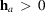International
Tables for
Crystallography
Volume B
Reciprocal space
Edited by U. Shmueli

International Tables for Crystallography (2010). Vol. B, ch. 1.4, p. 116   | 1 | 2 |

## Section 1.4.2.3. Symmetry factors for space-group-specific Fourier summations

U. Shmuelia

#### 1.4.2.3. Symmetry factors for space-group-specific Fourier summations

| top | pdf |

The weighted reciprocal lattice, with weights taken as the structure factors, is synonymous with the discrete space of the coefficients of a Fourier expansion of the electron density, or the Fourier space (F space) of the latter. Accordingly, the asymmetric unit of the Fourier space can be defined as the subset of structure factors within which the relationship (1.4.2.3)does not hold – except at special positions in the reciprocal lattice. If the point group of the crystal is of order g, this is also the order of the corresponding factor-group representation of the space group (IT A, 1983) and there exist g relationships of the form of (1.4.2.3):We can thus decompose the summation in (1.4.2.1)into g sums, each extending over an asymmetric unit of the F space. It must be kept in mind, however, that some classes of reciprocal-lattice vectors may be common to more than one asymmetric unit, and thus each reciprocal-lattice point will be assigned an occupancy factor, denoted by, such thatfor a general position andfor a special one, whereis the multiplicity – or the order of the point group that leaves h unchanged. Equation (1.4.2.1)can now be rewritten aswhere the inner summation in (1.4.2.9)extends over the reference asymmetric unit of the Fourier space, which is associated with the identity operation of the space group. Substituting from (1.4.2.8)for, and interchanging the order of the summations in (1.4.2.9), we obtainwhereandThe symmetry factors A and B are well known as geometric or trigonometric structure factors and a considerable part of Volume I of IT (1952)is dedicated to their tabulation. Their formal association with the structure factor – following from direct-space arguments – is closely related to that shown in equation (1.4.2.11)(see Section 1.4.2.4). Simplified trigonometric expressions for A and B are given in Tables A1.4.3.1–A1.4.3.7in Appendix A1.4.3for all the two- and three-dimensional crystallographic space groups, and for all the parities of hkl for which A and B assume different functional forms. These expressions are there given for general reflections and can also be used for special ones, provided the occupancy factorshave been properly accounted for.

Equation (1.4.2.11)is quite general and can, of course, be applied to noncentrosymmetric Fourier summations, without neglect of dispersion. Further simplifications are obtained in the centrosymmetric case, when the space-group origin is chosen at a centre of symmetry, and in the noncentrosymmetric case, when dispersion is neglected. In each of the latter two cases the summation overis restricted to reciprocal-lattice vectors that are not related by real or apparent inversion (denoted by), and we obtainandfor the dispersionless centrosymmetric and noncentrosymmetric cases, respectively.

### References

International Tables for Crystallography (1983). Vol. A, Space-Group Symmetry, edited by Th. Hahn. Dordrecht: Reidel.
International Tables for X-ray Crystallography (1952). Vol. I, Symmetry Groups, edited by N. F. M. Henry & K. Lonsdale. Birmingham: Kynoch Press.